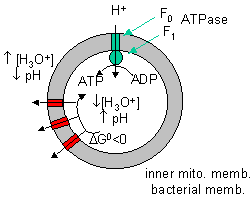# C11. Proton Gradient Collapse and ATP synthesis - Thermodynamics

$$\newcommand{\vecs}{\overset { \rightharpoonup} {\mathbf{#1}} }$$ $$\newcommand{\vecd}{\overset{-\!-\!\rightharpoonup}{\vphantom{a}\smash {#1}}}$$$$\newcommand{\id}{\mathrm{id}}$$ $$\newcommand{\Span}{\mathrm{span}}$$ $$\newcommand{\kernel}{\mathrm{null}\,}$$ $$\newcommand{\range}{\mathrm{range}\,}$$ $$\newcommand{\RealPart}{\mathrm{Re}}$$ $$\newcommand{\ImaginaryPart}{\mathrm{Im}}$$ $$\newcommand{\Argument}{\mathrm{Arg}}$$ $$\newcommand{\norm}{\| #1 \|}$$ $$\newcommand{\inner}{\langle #1, #2 \rangle}$$ $$\newcommand{\Span}{\mathrm{span}}$$ $$\newcommand{\id}{\mathrm{id}}$$ $$\newcommand{\Span}{\mathrm{span}}$$ $$\newcommand{\kernel}{\mathrm{null}\,}$$ $$\newcommand{\range}{\mathrm{range}\,}$$ $$\newcommand{\RealPart}{\mathrm{Re}}$$ $$\newcommand{\ImaginaryPart}{\mathrm{Im}}$$ $$\newcommand{\Argument}{\mathrm{Arg}}$$ $$\newcommand{\norm}{\| #1 \|}$$ $$\newcommand{\inner}{\langle #1, #2 \rangle}$$ $$\newcommand{\Span}{\mathrm{span}}$$

Experimental evidence shows that it can. The FoF1ATPase complex can be removed from membranes and placed in a liposome into which ADP and Pi have been encapsulated. The pH of the outside of the vesicles is then lowered several pH units. Under these circumstances, ATP is generated inside the vesicle proving that a gradient alone can drive its synthesis.

Mathematical analyses show that it can as well. Consider a typical pH gradient (-1.4 pH units) across the inner membrane of respiring mitochondria (with the outside having a lower pH than inside making the inside more depleted in protons). Clearly there is a chemical potential difference in protons across the membrane. However, another factor determines the thermodynamic driving force for proton translocation across the membrane. A transmembrane potential exists across the inner membrane of the mitochondria, as it does across most membranes. The source of the membrane potential will be discussed in signal transduction chapter. The inside is more negative than the outside, giving the membrane a transmembrane electrical potential. of about -0.14 V. Clearly, protons would be attracted to the other side of the membrane (into the matrix) by this potential difference, which then augments the chemical potential difference as well. A simple mathematical derivation shows that indeed, a proton gradient can supply enough energy for ATP synthesis, especially when coupled to a transmembrane electrical potential.

Figure: A simple mathematical derivationThe sum of the electrical and chemical potentials are called the electrochemical potential, which when divided by nF gives the proton motive force.

Note: In the above discussion, we dealt with two different proton translocating methods:

Figure: two different proton translocating methods1. Complex I, III, and IV, which couple uphill proton movement (from the higher pH matrix to the lower pH intermembrane space) to oxidation (NADH + O2 to NAD+ + H2O).
2. Downhill movement of protons through F0F1 ATPase which couples to ATP synthesis by the enzyme.